Area of Path and Cross roads - Worksheet

Chapter 9 Class 7 Perimeter and Area
Serial order wise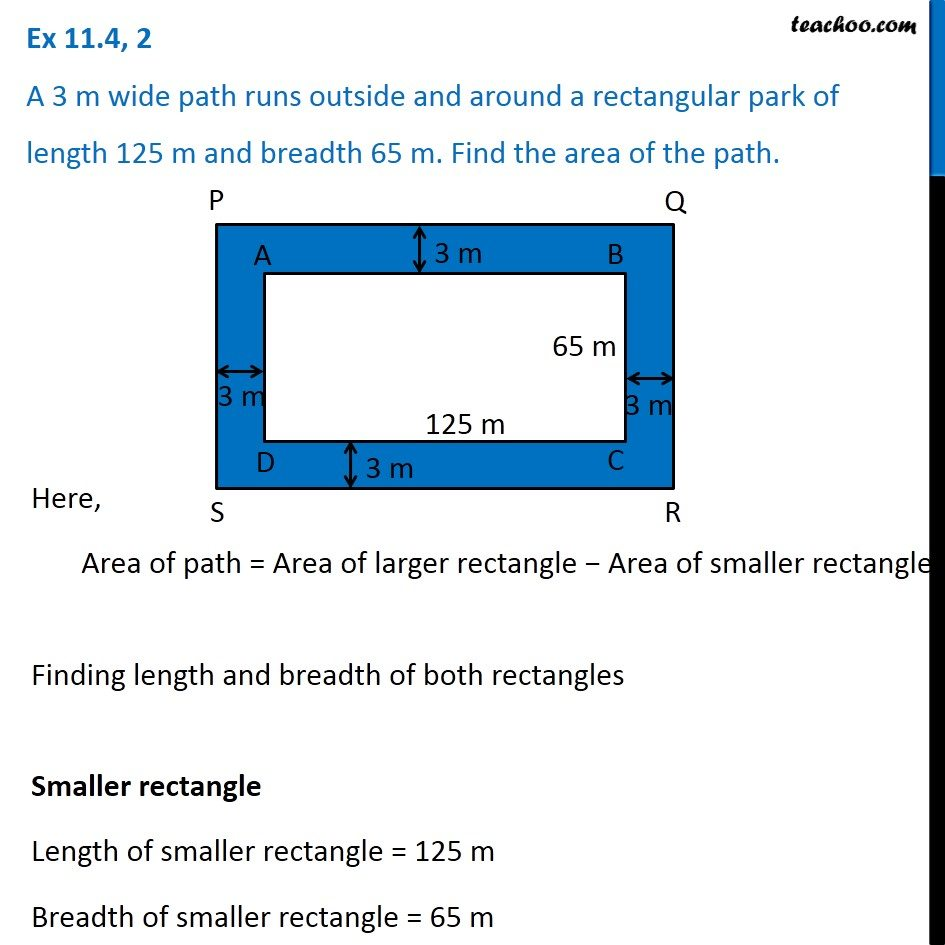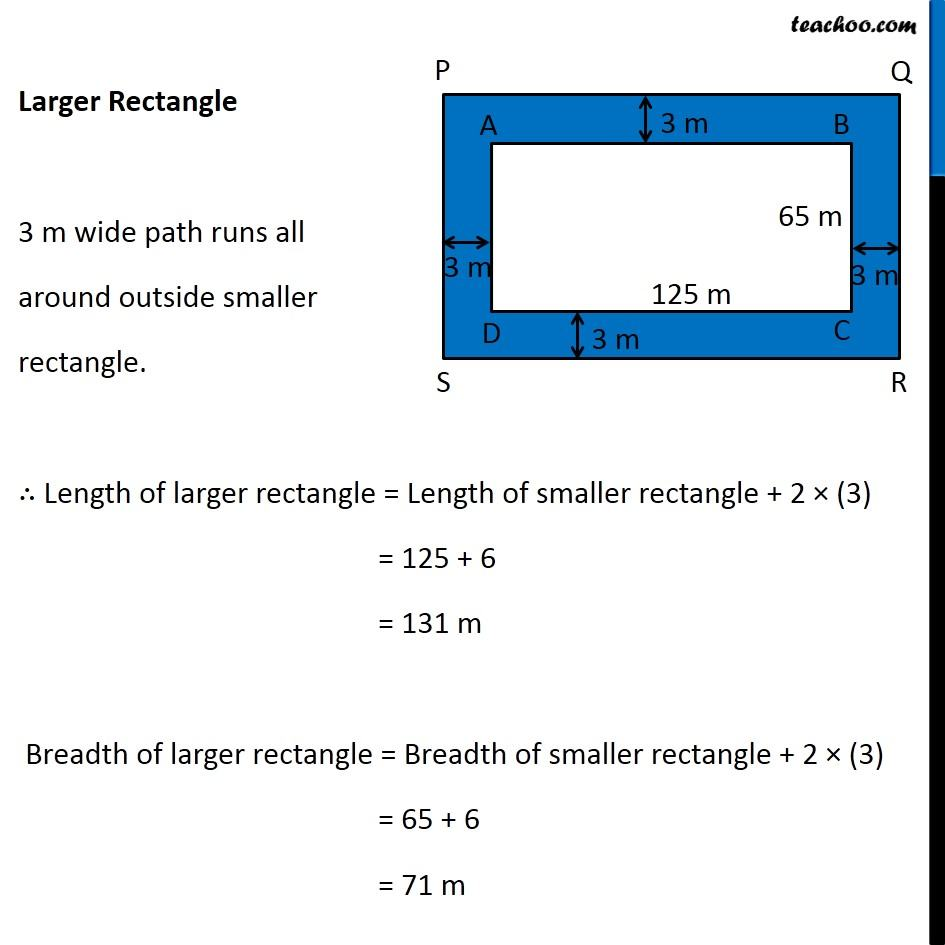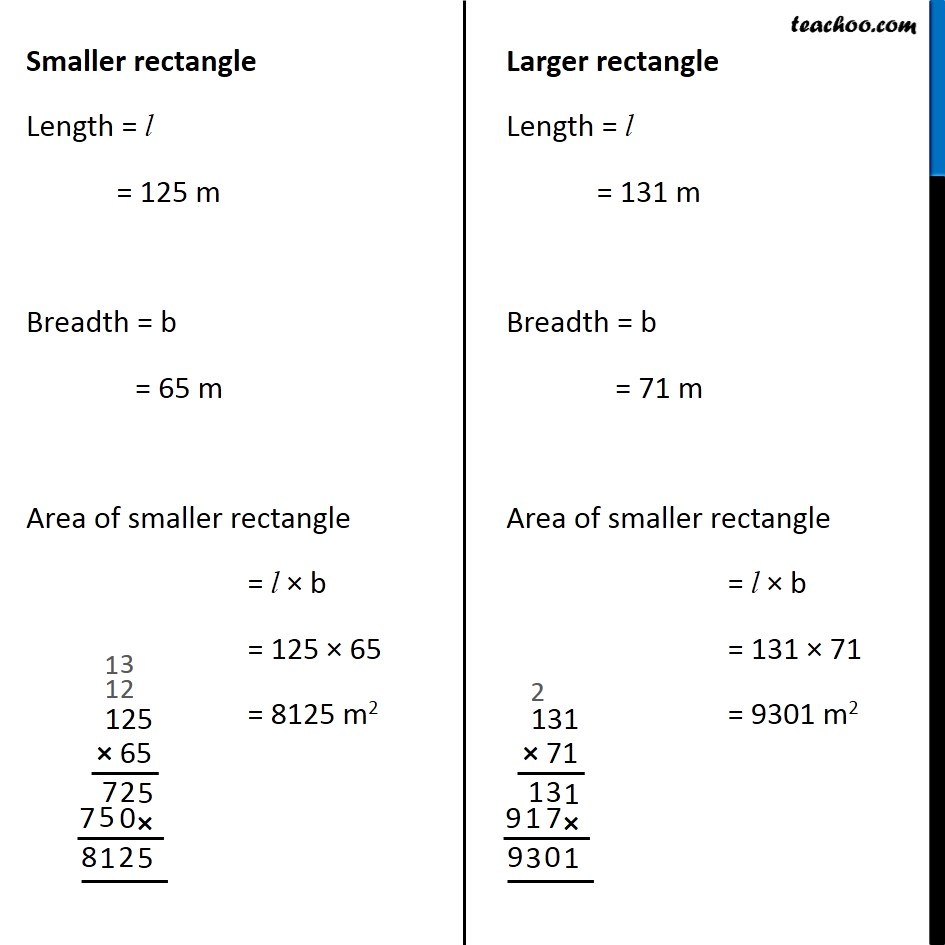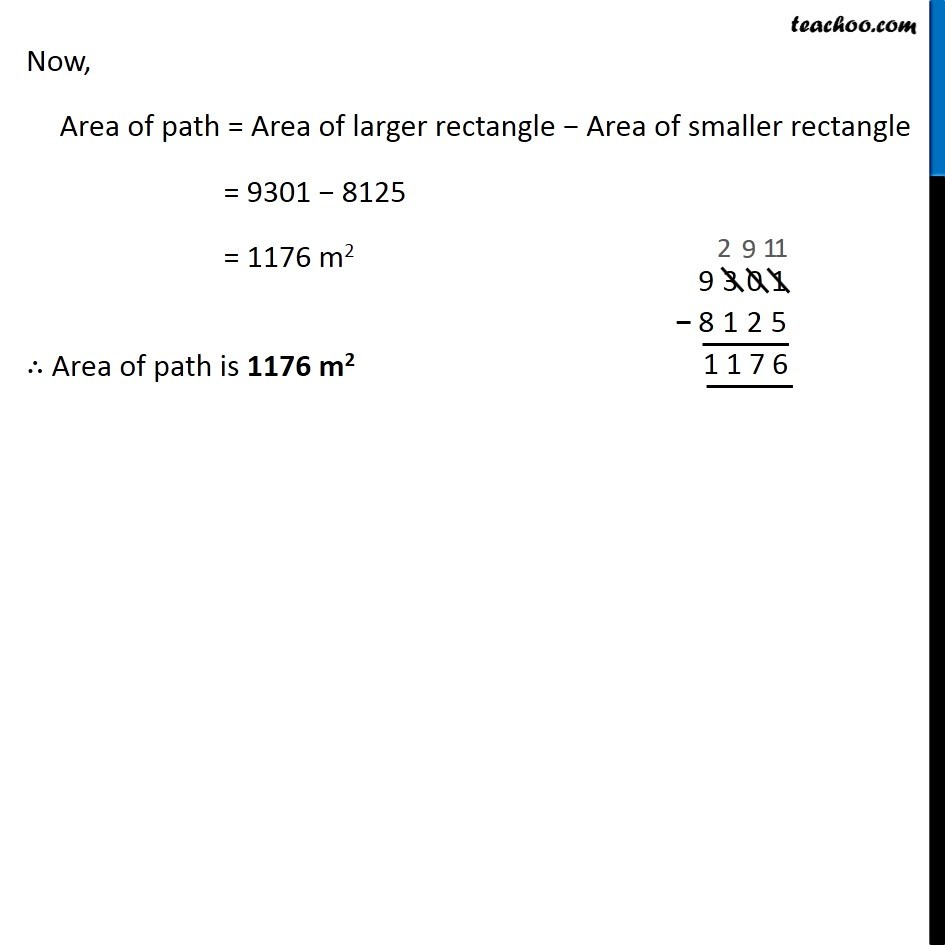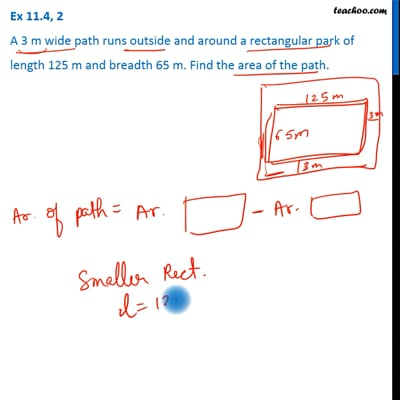This video is only available for Teachoo black users

Learn in your speed, with individual attention - Teachoo Maths 1-on-1 Class

### Transcript

Question 2 A 3 m wide path runs outside and around a rectangular park of length 125 m and breadth 65 m. Find the area of the path. Here, Area of path = Area of larger rectangle − Area of smaller rectangle Finding length and breadth of both rectangles Smaller rectangle Length of smaller rectangle = 125 m Breadth of smaller rectangle = 65 m Larger Rectangle 3 m wide path runs all around outside smaller rectangle. ∴ Length of larger rectangle = Length of smaller rectangle + 2 × (3) = 125 + 6 = 131 m Breadth of larger rectangle = Breadth of smaller rectangle + 2 × (3) = 65 + 6 = 71 m Smaller rectangle Length = l = 125 m Breadth = b = 65 m Area of smaller rectangle = l × b = 125 × 65 = 8125 m2 Larger rectangle Length = l = 131 m Breadth = b = 71 m Area of smaller rectangle = l × b = 131 × 71 = 9301 m2 Now, Area of path = Area of larger rectangle − Area of smaller rectangle = 9301 − 8125 = 1176 m2 ∴ Area of path is 1176 m2